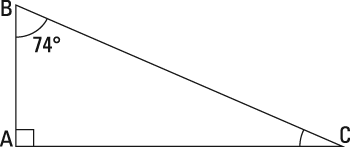##### ASVAB AFQT: 1,001 Practice Questions For DummiesTriangles are pretty common in real life, so you should expect to see triangle problems on the Mathematics Knowledge subtest on the ASVAB. In the following practice questions, you have to find an interior angle of a triangle, and then use algebra to solve for x in a congruent triangle.

## Practice questions

1. Find the measure of angle C:A. 106 degrees B. 16 degrees C. 36 degrees D. 30 degrees
2. Find x:

A. 10 B. 5 C. 4 D. 8

1. The correct answer is Choice (B). The sum of all angles in a triangle is 180 degrees, so fill in the values you know using A + B + C = 180 degrees and let x represent the angle you don't know (note that angle A is a right angle, so it's 90 degrees):

2. The correct answer is Choice (A). The triangle has two congruent sides (that's what the hash marks represent). That means the two sides are equal. Set their values equal to each other and solve for x: